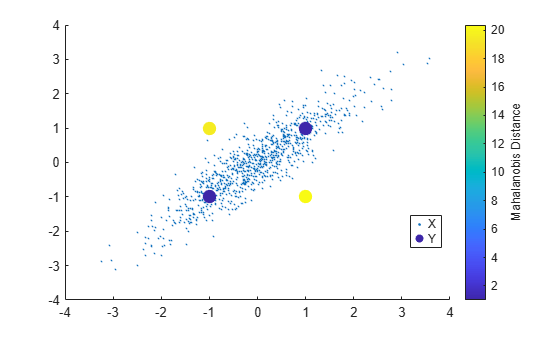# mahal

Mahalanobis distance to reference samples

## Syntax

``d2 = mahal(Y,X)``

## Description

example

````d2 = mahal(Y,X)` returns the squared Mahalanobis distance of each observation in `Y` to the reference samples in `X`.```

## Examples

collapse all

Generate a correlated bivariate sample data set.

```rng('default') % For reproducibility X = mvnrnd([0;0],[1 .9;.9 1],1000);```

Specify four observations that are equidistant from the mean of `X` in Euclidean distance.

`Y = [1 1;1 -1;-1 1;-1 -1];`

Compute the Mahalanobis distance of each observation in `Y` to the reference samples in `X`.

`d2_mahal = mahal(Y,X)`
```d2_mahal = 4×1 1.1095 20.3632 19.5939 1.0137 ```

Compute the squared Euclidean distance of each observation in `Y` from the mean of `X` .

`d2_Euclidean = sum((Y-mean(X)).^2,2)`
```d2_Euclidean = 4×1 2.0931 2.0399 1.9625 1.9094 ```

Plot `X` and `Y` by using `scatter` and use marker color to visualize the Mahalanobis distance of `Y` to the reference samples in `X`.

```scatter(X(:,1),X(:,2),10,'.') % Scatter plot with points of size 10 hold on scatter(Y(:,1),Y(:,2),100,d2_mahal,'o','filled') hb = colorbar; ylabel(hb,'Mahalanobis Distance') legend('X','Y','Location','best')```All observations in `Y` (`[1,1]`, `[-1,-1,]`, `[1,-1]`, and `[-1,1]`) are equidistant from the mean of `X` in Euclidean distance. However, `[1,1]` and `[-1,-1]` are much closer to X than `[1,-1]` and `[-1,1]` in Mahalanobis distance. Because Mahalanobis distance considers the covariance of the data and the scales of the different variables, it is useful for detecting outliers.

## Input Arguments

collapse all

Data, specified as an n-by-m numeric matrix, where n is the number of observations and m is the number of variables in each observation.

`X` and `Y` must have the same number of columns, but can have different numbers of rows.

Data Types: `single` | `double`

Reference samples, specified as a p-by-m numeric matrix, where p is the number of samples and m is the number of variables in each sample.

`X` and `Y` must have the same number of columns, but can have different numbers of rows. `X` must have more rows than columns.

Data Types: `single` | `double`

## Output Arguments

collapse all

Squared Mahalanobis distance of each observation in `Y` to the reference samples in `X`, returned as an n-by-1 numeric vector, where n is the number of observations in `X`.

collapse all

### Mahalanobis Distance

The Mahalanobis distance is a measure between a sample point and a distribution.

The Mahalanobis distance from a vector y to a distribution with mean μ and covariance Σ is

`$d=\sqrt{\left(y-\mu \right){\sum }^{-1}\left(y-\mu \right)\text{'}}.$`

This distance represents how far y is from the mean in number of standard deviations.

`mahal` returns the squared Mahalanobis distance d2 from an observation in `Y` to the reference samples in `X`. In the `mahal` function, μ and Σ are the sample mean and covariance of the reference samples, respectively.